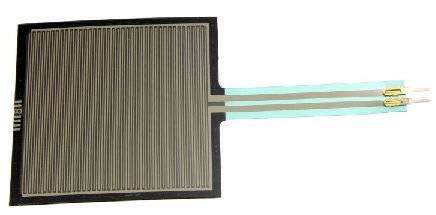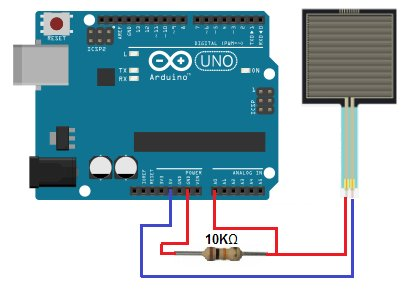How to Build a Simple Force Sensing Resistor (FSR) Circuit

In this article, we will go over how to connect a force sensing resistor, or force sensitive resistor, (FSR) to a circuit to build many different types of useful circuits with them.Force sensing resistors are variable resistors which change resistance according to the pressure or force applied to them. Under no force, the resistance is very high, pretty much infinite. When a light pressure is applied to the sensor, its resistance drops to about 100KΩ. When maximum pressure is applied, its resistance drops to about 200Ω.

So with no pressure or weight applied, the FSR circuit will be essentially open because its resistance is so high.

With a good amount of pressure applied, about 20lbs needed, the resistance drops dramatically and can go as low as 200Ω.

We will use the basic functioning principles that FSRs have to create a meaningful circuit.

We will wire an FSR to an arduino board. We wire a 10KΩ resistor in series with the force sensing resistor in order to create a voltage divider. One terminal of the FSR will connect to the 5-volt voltage supply of the arduino board and the other terminal will connect to ground. The 5 volts of voltage will be divided based on the resistance of the fixed resistor and the FSR. The fixed resistor will always stay 10KΩ. However, the FSR serves as the variable resistor. It will change resistance based on the pressure applied to its surface. When there is no pressure applied, its resistance is very high, so most of the voltage falls across it rather than the 10KΩ resistor. When it is pressed against with maximum pressure, its resistance falls to near 200Ω, so most of the voltage falls across the 10KΩ resistor and not the FSR.

READ  Schematic Entry and PCB layout

Based on these voltage divisions, we can get readings of how much pressure it is being applied to the FSR. If a high voltage is across it, we know that it barely has any pressure applied to it. If a low voltage is across it, then it has a lot of pressure applied to it. The arduino serves as a microcontroller that can give us “pressure” readings of the FSR circuit.

This type of circuit could be useful for a wide range of real-life scenarios. Think of a car seat that is equipped with a seatbelt light on the car dashboard. How does it know to light the dashboard, indicating that the passenger seatbelt is not on? How does it know that a passenger is present in the passenger seat or not? It knows because it detects weight. If it detects weight and the seatbelt is not on, the seatbelt LED on the dashboard lights up, indicating that the seatbelt is not on.

We could apply this circuit that we’re going to build for a situation such as the car seatbelt lights. If our FSR has a very low voltage, this indicates that a lot of pressure is being applied to it. This would indicate that a passenger is present in the seat. If this is the case and the seatbelt is not on, this would trigger the passenger seatlight light to turn on in the dashboard.

Parts Needed for the FSR Circuit

• Force sensing resistor
• 10KΩ Resistor
• Arduino Board

The force sensing resistor can be obtained from many different online retailers. It can be bought in a circular form or square form. A great selection can be found at digikey at the following link: Digikey- Force sensing resistors.

We will use 5 volts of power from the 5V terminal of the arduino.

FSR Circuit

The circuit we will build is shown below:Again, the 10KΩ resistor is in series with the FSR. This forms a voltage divider circuit with the 5 volts powering it. This 5 volts will be divided between the 2 components, based on the resistance of each of the components. Based on the voltage across the components, the arduino board can read how much pressure is being applied to the FSR.

For more detail: How to Build a Simple Force Sensing Resistor (FSR) Circuit

This Post / Project can also be found using search terms:

• how to build a pedometer with fsr with arduino
• what board to use in force sensing circuit
• force sensing resistor projects
• fsr sensor circuit